Intended for healthcare professionals
Home/11. Correlation and regression

# 11. Correlation and regression

The word correlation is used in everyday life to denote some form of association. We might say that we have noticed a correlation between foggy days and attacks of wheeziness. However, in statistical terms we use correlation to denote association between two quantitative variables. We also assume that the association is linear, that one variable increases or decreases a fixed amount for a unit increase or decrease in the other. The other technique that is often used in these circumstances is regression, which involves estimating the best straight line to summarise the association.

## Correlation coefficient

The degree of association is measured by a correlation coefficient, denoted by r. It is sometimes called Pearson’s correlation coefficient after its originator and is a measure of linear association. If a curved line is needed to express the relationship, other and more complicated measures of the correlation must be used.
The correlation coefficient is measured on a scale that varies from + 1 through 0 to – 1. Complete correlation between two variables is expressed by either + 1 or -1. When one variable increases as the other increases the correlation is positive; when one decreases as the other increases it is negative. Complete absence of correlation is represented by 0. Figure 11.1 gives some graphical representations of correlation.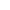Figure 11.1 Correlation illustrated.

## Looking at data: scatter diagrams

When an investigator has collected two series of observations and wishes to see whether there is a relationship between them, he or she should first construct a scatter diagram. The vertical scale represents one set of measurements and the horizontal scale the other. If one set of observations consists of experimental results and the other consists of a time scale or observed classification of some kind, it is usual to put the experimental results on the vertical axis. These represent what is called the “dependent variable”. The “independent variable”, such as time or height or some other observed classification, is measured along the horizontal axis, or baseline.
The words “independent” and “dependent” could puzzle the beginner because it is sometimes not clear what is dependent on what. This confusion is a triumph of common sense over misleading terminology, because often each variable is dependent on some third variable, which may or may not be mentioned. It is reasonable, for instance, to think of the height of children as dependent on age rather than the converse but consider a positive correlation between mean tar yield and nicotine yield of certain brands of cigarette.’ The nicotine liberated is unlikely to have its origin in the tar: both vary in parallel with some other factor or factors in the composition of the cigarettes. The yield of the one does not seem to be “dependent” on the other in the sense that, on average, the height of a child depends on his age. In such cases it often does not matter which scale is put on which axis of the scatter diagram. However, if the intention is to make inferences about one variable from the other, the observations from which the inferences are to be made are usually put on the baseline. As a further example, a plot of monthly deaths from heart disease against monthly sales of ice cream would show a negative association. However, it is hardly likely that eating ice cream protects from heart disease! It is simply that the mortality rate from heart disease is inversely related – and ice cream consumption positively related – to a third factor, namely environmental temperature.

## Calculation of the correlation coefficient

A paediatric registrar has measured the pulmonary anatomical dead space (in ml) and height (in cm) of 15 children. The data are given in table 11.1 and the scatter diagram shown in figure 11.2 Each dot represents one child, and it is placed at the point corresponding to the measurement of the height (horizontal axis) and the dead space (vertical axis). The registrar now inspects the pattern to see whether it seems likely that the area covered by the dots centres on a straight line or whether a curved line is needed. In this case the paediatrician decides that a straight line can adequately describe the general trend of the dots. His next step will therefore be to calculate the correlation coefficient.When making the scatter diagram (figure 11.2 ) to show the heights and pulmonary anatomical dead spaces in the 15 children, the paediatrician set out figures as in columns (1), (2), and (3) of table 11.1 . It is helpful to arrange the observations in serial order of the independent variable when one of the two variables is clearly identifiable as independent. The corresponding figures for the dependent variable can then be examined in relation to the increasing series for the independent variable. In this way we get the same picture, but in numerical form, as appears in the scatter diagram.Figure 11.2 Scatter diagram of relation in 15 children between height and pulmonary anatomical dead space.
The calculation of the correlation coefficient is as follows, with x representing the values of the independent variable (in this case height) and y representing the values of the dependent variable (in this case anatomical dead space). The formula to be used is:which can be shown to be equal to: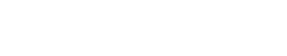## Calculator procedure

Find the mean and standard deviation of x, as described in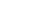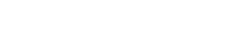Find the mean and standard deviation of y:Subtract 1 from n and multiply by SD(x) and SD(y), (n – 1)SD(x)SD(y)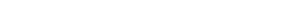This gives us the denominator of the formula. (Remember to exit from “Stat” mode.)
For the numerator multiply each value of x by the corresponding value of y, add these values together and store them.
110 x 44 = Min
116 x 31 = M+
etc.
This stores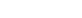in memory. Subtract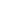MR – 15 x 144.6 x 66.93 (5426.6)
Finally divide the numerator by the denominator.
r = 5426.6/6412.0609 = 0.846.
The correlation coefficient of 0.846 indicates a strong positive correlation between size of pulmonary anatomical dead space and height of child. But in interpreting correlation it is important to remember that correlation is not causation. There may or may not be a causative connection between the two correlated variables. Moreover, if there is a connection it may be indirect.
A part of the variation in one of the variables (as measured by its variance) can be thought of as being due to its relationship with the other variable and another part as due to undetermined (often “random”) causes. The part due to the dependence of one variable on the other is measured by Rho . For these data Rho= 0.716 so we can say that 72% of the variation between children in size of the anatomical dead space is accounted for by the height of the child. If we wish to label the strength of the association, for absolute values of r, 0-0.19 is regarded as very weak, 0.2-0.39 as weak, 0.40-0.59 as moderate, 0.6-0.79 as strong and 0.8-1 as very strong correlation, but these are rather arbitrary limits, and the context of the results should be considered.

## Significance test

To test whether the association is merely apparent, and might have arisen by chance use the t test in the following calculation:is entered at n – 2 degrees of freedom.
For example, the correlation coefficient for these data was 0.846.
The number of pairs of observations was 15. Applying equation 11.1, we have:Entering table B at 15 – 2 = 13 degrees of freedom we find that at t = 5.72, P < 0.001 so the correlation coefficient may be regarded as highly significant. Thus (as could be seen immediately from the scatter plot) we have a very strong correlation between dead space and height which is most unlikely to have arisen by chance.
The assumptions governing this test are:
1. That both variables are plausibly Normally distributed.
2. That there is a linear relationship between them.
3. The null hypothesis is that there is no association between them.
The test should not be used for comparing two methods of measuring the same quantity, such as two methods of measuring peak expiratory flow rate. Its use in this way appears to be a common mistake, with a significant result being interpreted as meaning that one method is equivalent to the other. The reasons have been extensively discussed(2) but it is worth recalling that a significant result tells us little about the strength of a relationship. From the formula it should be clear that with even with a very weak relationship (say r = 0.1) we would get a significant result with a large enough sample (say n over 1000).

## Spearman rank correlation

A plot of the data may reveal outlying points well away from the main body of the data, which could unduly influence the calculation of the correlation coefficient. Alternatively the variables may be quantitative discrete such as a mole count, or ordered categorical such as a pain score. A non-parametric procedure, due to Spearman, is to replace the observations by their ranks in the calculation of the correlation coefficient.
This results in a simple formula for Spearman’s rank correlation, Rho.where d is the difference in the ranks of the two variables for a given individual. Thus we can derive table 11.2 from the data in table 11.1 .From this we get thatIn this case the value is very close to that of the Pearson correlation coefficient. For n> 10, the Spearman rank correlation coefficient can be tested for significance using the t test given earlier.

## The regression equation

Correlation describes the strength of an association between two variables, and is completely symmetrical, the correlation between A and B is the same as the correlation between B and A. However, if the two variables are related it means that when one changes by a certain amount the other changes on an average by a certain amount. For instance, in the children described earlier greater height is associated, on average, with greater anatomical dead Space. If y represents the dependent variable and x the independent variable, this relationship is described as the regression of y on x.
The relationship can be represented by a simple equation called the regression equation. In this context “regression” (the term is a historical anomaly) simply means that the average value of y is a “function” of x, that is, it changes with x.
The regression equation representing how much y changes with any given change of x can be used to construct a regression line on a scatter diagram, and in the simplest case this is assumed to be a straight line. The direction in which the line slopes depends on whether the correlation is positive or negative. When the two sets of observations increase or decrease together (positive) the line slopes upwards from left to right; when one set decreases as the other increases the line slopes downwards from left to right. As the line must be straight, it will probably pass through few, if any, of the dots. Given that the association is well described by a straight line we have to define two features of the line if we are to place it correctly on the diagram. The first of these is its distance above the baseline; the second is its slope. They are expressed in the following regression equation :With this equation we can find a series of values of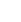the variable, that correspond to each of a series of values of x, the independent variable. The parameters α and β have to be estimated from the data. The parameter signifies the distance above the baseline at which the regression line cuts the vertical (y) axis; that is, when y = 0. The parameter β (the regression coefficient) signifies the amount by which change in x must be multiplied to give the corresponding average change in y, or the amount y changes for a unit increase in x. In this way it represents the degree to which the line slopes upwards or downwards.
The regression equation is often more useful than the correlation coefficient. It enables us to predict y from x and gives us a better summary of the relationship between the two variables. If, for a particular value of x, x i, the regression equation predicts a value of y fit , the prediction error is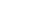. It can easily be shown that any straight line passing through the mean values x and y will give a total prediction error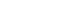of zero because the positive and negative terms exactly cancel. To remove the negative signs we square the differences and the regression equation chosen to minimise the sum of squares of the prediction errors,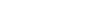We denote the sample estimates of Alpha and Beta by a and b. It can be shown that the one straight line that minimises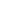, the least squares estimate, is given byand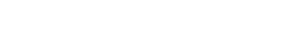it can be shown thatwhich is of use because we have calculated all the components of equation (11.2) in the calculation of the correlation coefficient.
The calculation of the correlation coefficient on the data in table 11.2 gave the following: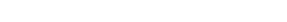Applying these figures to the formulae for the regression coefficients, we have: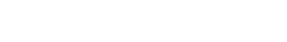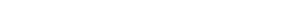Therefore, in this case, the equation for the regression of y on x becomes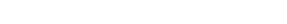This means that, on average, for every increase in height of 1 cm the increase in anatomical dead space is 1.033 ml over the range of measurements made.
The line representing the equation is shown superimposed on the scatter diagram of the data in figure 11.2. The way to draw the line is to take three values of x, one on the left side of the scatter diagram, one in the middle and one on the right, and substitute these in the equation, as follows:
If x = 110, y = (1.033 x 110) – 82.4 = 31.2
If x = 140, y = (1.033 x 140) – 82.4 = 62.2
If x = 170, y = (1.033 x 170) – 82.4 = 93.2
Although two points are enough to define the line, three are better as a check. Having put them on a scatter diagram, we simply draw the line through them.Figure 11.3 Regression line drawn on scatter diagram relating height and pulmonaiy anatomical dead space in 15 children
The standard error of the slope SE(b) is given by:where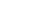is the residual standard deviation, given by:This can be shown to be algebraically equal to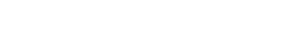We already have to hand all of the terms in this expression. Thusis the square root of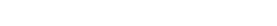. The denominator of (11.3) is 72.4680. Thus SE(b) = 13.08445/72.4680 = 0.18055.
We can test whether the slope is significantly different from zero by:
t = b/SE(b) = 1.033/0.18055 = 5.72.
Again, this has n – 2 = 15 – 2 = 13 degrees of freedom. The assumptions governing this test are:
1. That the prediction errors are approximately Normally distributed. Note this does not mean that the x or y variables have to be Normally distributed.
2. That the relationship between the two variables is linear.
3. That the scatter of points about the line is approximately constant – we would not wish the variability of the dependent variable to be growing as the independent variable increases. If this is the case try taking logarithms of both the x and y variables.
Note that the test of significance for the slope gives exactly the same value of P as the test of significance for the correlation coefficient. Although the two tests are derived differently, they are algebraically equivalent, which makes intuitive sense.
We can obtain a 95% confidence interval for b fromwhere the tstatistic from has 13 degrees of freedom, and is equal to 2.160.
Thus the 95% confidence interval is
l.033 – 2.160 x 0.18055 to l.033 + 2.160 x 0.18055 = 0.643 to 1.422.
Regression lines give us useful information about the data they are collected from. They show how one variable changes on average with another, and they can be used to find out what one variable is likely to be when we know the other – provided that we ask this question within the limits of the scatter diagram. To project the line at either end – to extrapolate – is always risky because the relationship between x and y may change or some kind of cut off point may exist. For instance, a regression line might be drawn relating the chronological age of some children to their bone age, and it might be a straight line between, say, the ages of 5 and 10 years, but to project it up to the age of 30 would clearly lead to error. Computer packages will often produce the intercept from a regression equation, with no warning that it may be totally meaningless. Consider a regression of blood pressure against age in middle aged men. The regression coefficient is often positive, indicating that blood pressure increases with age. The intercept is often close to zero, but it would be wrong to conclude that this is a reliable estimate of the blood pressure in newly born male infants!

More than one independent variable is possible – in such a case the method is known as multiple regression. (3,4 )This is the most versatile of statistical methods and can be used in many situations. Examples include: to allow for more than one predictor, age as well as height in the above example; to allow for covariates – in a clinical trial the dependent variable may be outcome after treatment, the first independent variable can be binary, 0 for placebo and 1 for active treatment and the second independent variable may be a baseline variable, measured before treatment, but likely to affect outcome.

## Common questions

If two variables are correlated are they causally related?
It is a common error to confuse correlation and causation. All that correlation shows is that the two variables are associated. There may be a third variable, a confounding variable that is related to both of them. For example, monthly deaths by drowning and monthly sales of ice-cream are positively correlated, but no-one would say the relationship was causal!
How do I test the assumptions underlying linear regression?
Firstly always look at the scatter plot and ask, is it linear? Having obtained the regression equation, calculate the residuals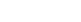A histogram of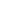will reveal departures from Normality and a plot ofversuswill reveal whether the residuals increase in size asincreases.

## References

1. Russell MAH, Cole PY, Idle MS, Adams L. Carbon monoxide yields of cigarettes and their relation to nicotine yield and type of filter. BMJ 1975; 3:713.
2. Bland JM, Altman DG. Statistical methods for assessing agreement between two methods of clinical measurement. Lancet 1986; i:307-10.
3. Brown RA, Swanson-Beck J. Medical Statistics on Personal Computers , 2nd edn. London: BMJ Publishing Group, 1993.
4. Armitage P, Berry G. In: Statistical Methods in Medical Research , 3rd edn. Oxford: Blackwell Scientific Publications, 1994:312-41.

## Exercises

11.1 A study was carried out into the attendance rate at a hospital of people in 16 different geographical areas, over a fixed period of time. The distance of the centre from the hospital of each area was measured in miles. The results were as follows:
(1) 21%, 6.8; (2) 12%, 10.3; (3) 30%, 1.7; (4) 8%, 14.2; (5) 10%, 8.8; (6) 26%, 5.8; (7) 42%, 2.1; (8) 31%, 3.3; (9) 21%, 4.3; (10) 15%, 9.0; (11) 19%, 3.2; (12) 6%, 12.7; (13) 18%, 8.2; (14) 12%, 7.0; (15) 23%, 5.1; (16) 34%, 4.1.
What is the correlation coefficient between the attendance rate and mean distance of the geographical area?
11.2 Find the Spearman rank correlation for the data given in 11.1.
11.3 If the values of x from the data in 11.1 represent mean distance of the area from the hospital and values of y represent attendance rates, what is the equation for the regression of y on x? What does it mean?
11.4 Find the standard error and 95% confidence interval for the slope

## UK Jobs

Quantock Vale Surgery: GP partner (4 Sessions) in Taunton - Quantock Vale Surgery